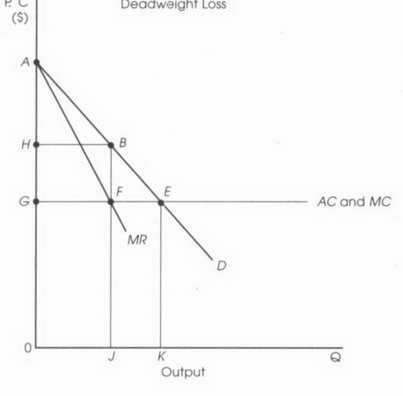# AP Microeconomics Practice Test 9

### Test Information12 questions14 minutes

1. Which of the following is a correct statement?

2. Which of the following correctly identifies the condition that explains inefficiency for firms in a monopolistically competitive industry?

3.The original consumer surplus (before monopoly) is

4.The dead-weight loss as the result of monopoly is

5.The reduced consumer surplus as the result of monopoly is

6. If the price of Product X increases, which of the following will be true of the quantity demanded, total revenue, and the price elasticity of demand?

Quantity demanded      Total revenue      Price elasticity of demand

7. If the price of one good (A) increases and the quantity demanded of another good (B) increases, the two goods are

8. If, as the price of a particular good increases, the added satisfaction of each additional unit of this good for the consumer decreases, which of the following correctly identifies the concept?

9. If, for each additional unit of a variable input, the increases in output become smaller, which of the following correctly identifies the concept?

10. Which of the following is correct about the demand for labor?

11. The greater (higher) the wage elasticity of demand, the

12. Which of the following is an example of a transfer earning?